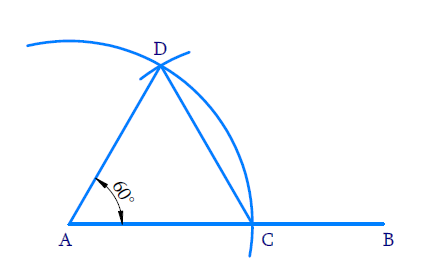# Ex.11.1 Q5 Constructions Solution - NCERT Maths Class 9

Go back to  'Ex.11.1'

## Question

Construct an equilateral triangle, given its side and justify the construction.

## Text Solution

Reasoning:

An equilateral triangle has three equal sides and three angles equal to $$60^\circ$$.We know how to construct an angle of $$60^{\circ}$$ .

Steps of Construction:(i) Draw ray $$AB$$

(ii) With $$A$$ as center and radius equal to $$3\;\rm{cm}$$ draw an arc to cut ray $$AB$$ at $$C$$ such that $$AC= 3\;\rm{cm}$$

(iii) With $$C$$ as the center and radius equal to $$AC$$, draw an arc to intersect the initial arc at $$D$$.

(iv) Join $$AD$$ and $$AC$$.

Thus,Triangle $$ADC$$ is an equilateral triangle.

Justification:

$$\rm{AC = AD}$$ (By construction since the radius of the arc is the same)

$$\rm{AC = CD}$$  (By construction since the same radius was used again)

$$\therefore \rm{AC = AD = CD}$$

$$\therefore ADC$$ is an equilateral triangle

Learn from the best math teachers and top your exams

• Live one on one classroom and doubt clearing
• Practice worksheets in and after class for conceptual clarity
• Personalized curriculum to keep up with school